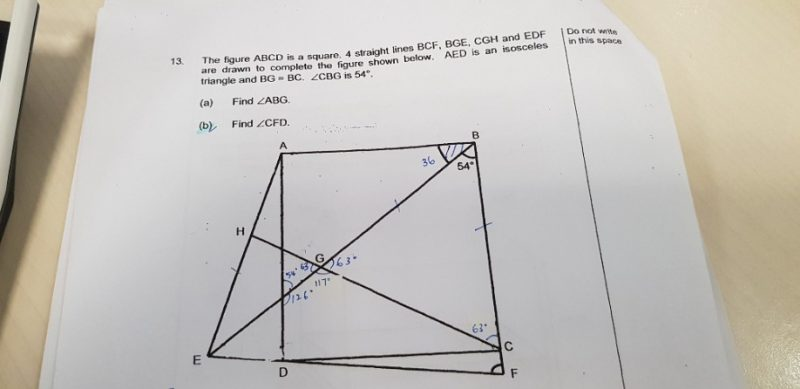# QuestionHi, can anyone help me part b pls. Thanks

1 Answer

# Answer

Suggested answer:

(b)  If AED is isosceles triangle, AE = AD

As ABCD is a square, AE = AD = AB, therefore AEB is also an isosceles triangle.

Angle EAB = (180 – 36 – 36) degree = 108 degree

Angle EAD = (108 – 90) degree = 18 degree

Angle ADE = (180 – 18)/2 degree = 81 degree

Angle CFD = angle ADE = 81 degree

1 Reply 0 Likes

May i know why angle CFD = angle ADE? Thanks

1 Reply 0 Likes

As ABCD is a square,  BCF // AD, angle ADF + angle CFD = 180 degree

0 Replies 0 Likes